## Main

In water, perturbations of the free surface manifest themselves by a small change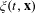of the water height. On a flat bottom, and in the absence of flow, linear perturbations are well described by superpositions of plane waves of definite frequency f (Hz) and wavevector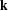(rad m−1). When surface waves propagate on a changing flow, the surface elevation is generally described by the sum of two contributions ξ = ξI + ξS, where ξI is the incident wave produced by a source, for example, a wave generator, while ξS is the scattered wave, generated by the interaction between the incident wave and the background flow. In this work, we are interested on the properties of this scattering on a draining vortex flow which is assumed to be axisymmetric and stationary. At the free surface, the velocity field is given in cylindrical coordinates by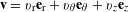.

Due to the symmetry, it is appropriate to describe ξI and ξS using polar coordinates (r, θ). Any wave ξ(t, r, θ) can be decomposed into partial waves10,14,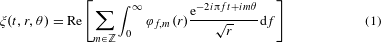where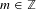is the azimuthal wavenumber and φf, m(r) denotes the radial part of the wave. Each component of this decomposition has a fixed angular momentum proportional to m, instead of a fixed wavevector. (To simplify notation, we drop the indices f, m in the following.) Since the background is stationary and axisymmetric, waves with different f and m propagate independently. Far from the centre of the vortex, the flow is very slow, and the radial part φ(r) becomes a sum of oscillatory solutions,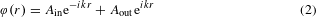where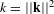is the wavevector norm. This describes the superposition of an inward wave of (complex) amplitude Ain propagating towards the vortex, and an outward wave propagating away from it with amplitude Aout. These coefficients are not independent. The Ain values, one for each f and m component, are fixed by the incident part ξI. If the incident wave is a plane wave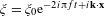, then the partial amplitudes are given by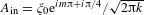. In other words, a plane wave is a superposition containing all azimuthal waves, something that we have exploited in our experiment. In contrast, Aout depends on the scattered part ξS, and how precisely the waves propagate in the centre and interact with the background vortex flow. In the limit of small amplitudes, there is a linear relation between the Ain and Aout values, and by the symmetries of the flow, different f and m decouple10,15.

This allows us to define the reflection coefficient at fixed f and m as the ratio between the outward (Jout) and inward (Jin) energy fluxes,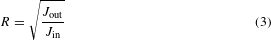In the linear approximation, the wave energy is a quadratic quantity in wave amplitude, and R is proportional to the amplitude ratio |Aout/Ain|.

If |R| < 1, the wave has lost energy during the scattering, and hence has undergone absorption. In this work we show experimentally that, under certain conditions, the reflection coefficient satisfies |R| > 1. We further argue that the amplified wave has extracted rotational energy from the vortex during the process.

We conducted our experiment in a rectangular water tank (length 3 m and width 1.5 m). Water is pumped continuously in from one end corner, and is drained through a hole (4 cm in diameter) in the middle. The water flows in a closed circuit. We first establish a stationary rotating draining flow by setting the flow rate of the pump to 37.5 ± 0.5 l min−1 and waiting until the depth (away from the vortex) is steady at 6.25 ± 0.05 cm. We then generate plane waves from one side of the tank, with an excitation frequency varying from 2.87 Hz to 4.11 Hz. On the side of the tank opposite the wave generator, we have placed an absorption beach (we have verified that the amount of reflection from the beach is below 5% in all experiments). We record the free surface with a high-speed three-dimensional air–fluid interface sensor. The sensor is a joint invention16 (patent no. DE 10 2015 001 365 A1) between The University of Nottingham and EnShape GmbH (Jena, Germany).

Using this data, we apply two filters. We first perform a Fourier transform in time, to single out the signal at the excitation frequency f0. This allows us to filter out the (stationary) background height, lying at f = 0, as well as the high-frequency noise. Moreover, we observe that the second harmonic, at 2f0, is also excited by the wave generator. This gives us an upper bound on the amount of nonlinearity of the system. In all experiments, the relative amplitude of the second harmonic compared to the fundamental stays below 14%. The obtained pattern shows a stationary wave of frequency f0 scattering on the vortex, which consists of the interfering superposition of the incident wave ξI with the scattered wave ξS. This pattern is shown on Fig. 1 for various frequencies, and looks very close to what was predicted on theoretical grounds for simple bathtub flow models10,17. We also observe that incident waves have more wavefronts on the upper half of the vortex in comparison with the lower half—see the various wave characteristics in Fig. 1a–f. This angular phase shift is analogous to the Aharonov–Bohm effect, and has been observed in previous water wave experiments18,19. Our detection method allows for a very clear visualization of this effect.Figure 1: Wave characteristics of the surface perturbation ξ, filtered at a single frequency, for six different frequencies.

The second filter is the polar Fourier transform, which selects a specific azimuthal wavenumber m, and allows the radial profile φ(r) to be determined. To extract the reflection coefficient, we use a windowed Fourier transform of the radial profile φ(r). The windowing is done on the interval [rmin, rmax]. When rmin is large enough, the radial profile φ contains two Fourier components (see equation (2)), one of negative k (inward wave), and one of positive k (outward wave). The ratio between their two amplitudes gives us the reflection coefficient (up to the energy correction, see Methods—Wave energy). To better resolve the two peaks, we have applied a Hamming window on the radial profile over the interval [rmin, rmax]. In all experiments, rmin 0.15 m, while rmax 0.39 m. We also point out that the minimum radius such that the radial profile reduces to equation (2) increases with m. With the size of our window, and the wavelength range of the experiment, we can resolve with confidence m = −2, −1,0,1,2.

On Fig. 2 we represent, for several azimuthal numbers m, the absolute value of the reflection coefficient R as a function of the frequency f. We observe two distinct behaviours, depending on the sign of m. Negative m values (waves counterrotating with the vortex) have a low reflection coefficient, which means that they are essentially absorbed in the vortex hole. On the other hand, positive m values have a reflection coefficient close to 1. In some cases this reflection is above 1, meaning that the corresponding mode has been amplified while scattering on the vortex. To confirm this amplification we have repeated the same experiment 15 times at a frequency f = 3.8 Hz and water height h0 = 6.25 ± 0.05 cm, for which the amplification was the highest. We present the result in Fig. 3. In this figure we clearly observe that the modes m = 1 and m = 2 are amplified by factors Rm=1 1.09 ± 0.03, and Rm=2 1.14 ± 0.08 respectively. In Figs 2 and 3, we have also shown the reflection coefficients obtained for a plane wave propagating on standing water of the same depth. Unlike what happens in the presence of a vortex, the reflection coefficients are all below 1 (within error bars). For low frequencies it is close to 1, meaning that the wave is propagating without losses, while for higher frequencies it decreases due to a loss of energy during the propagation—that is, damping.Figure 2: Reflection coefficients for various frequencies and various values of m.Figure 3: Reflection coefficients for different values of m, for the frequency f = 3.70 Hz (stars).

The origin of this amplification can be explained by the presence of negative energy waves20,21. Negative energy waves are excitations that lower the energy of the whole system (that is, background flow and excitation) instead of increasing it. In our case, the sign of the energy of a wave is given by the angular frequency in the fluid frame ωfluid. If the fluid rotates with an angular velocity Ω(r), in rad s−1, we have ωfluid = 2πf(r). At fixed frequency, when the fluid rotates fast enough, the energy becomes negative. If part of the wave is absorbed in the hole, carrying negative energy, the reflected part must come out with a higher positive energy to ensure conservation of the total energy2. Using particle imaging velocimetry (PIV), we have measured the velocity field of the vortex flow of our experiment. As we see on Fig. 4a, close to the centre, the angular velocity is fairly high, and the superradiant condition 2πf < is therefore satisfied for our frequency range.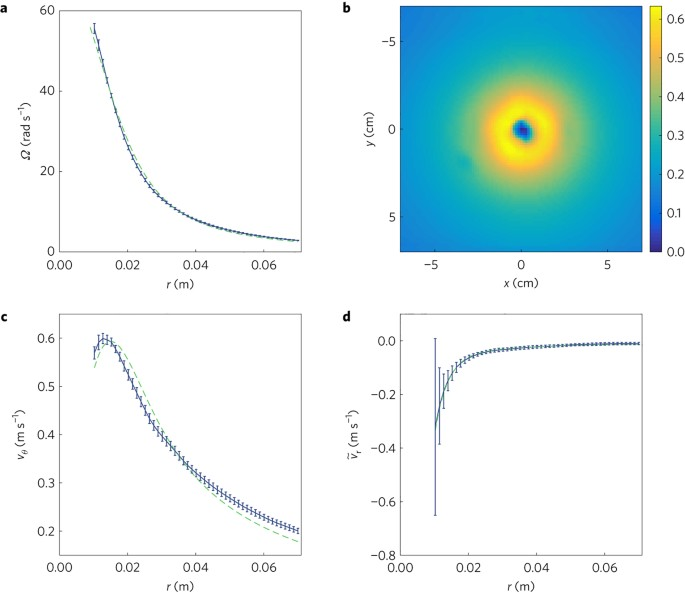Figure 4: PIV measurements of the velocity field averaged of 10 experiments.

Our experiment demonstrates that a wave scattering on a rotating vortex flow can carry away more energy than the incident wave brings in. Our results show that the phenomenon of superradiance is very robust and requires few ingredients to occur, namely high angular velocities, allowing for negative energy waves, and a mechanism to absorb these negative energies. For about half of the frequency range, our results confirm superradiant amplification despite a significant damping of the waves. The present experiment does not reveal the mechanism behind the absorption of the negative energies. The likely possibilities are that they are dissipated away in the vortex throat, in analogy to superradiant cylinders4,22, that they are trapped in the hole23 and unable to escape, similarly to what happens in black holes24,25, or a combination of both. A possible way to distinguish between the two in future experiments would be to measure the amount of energy going down the throat.

## Methods

### Wave energy.

To verify that the observed amplification increases the energy of the wave, we compare the energy current of the inward wave with respect to the outward wave. Since energy is transported by the group velocity vg, the energy current is given by J = gωfluid−1vg |A|2/f (up to the factor 1/f, this is the wave action, an adiabatic invariant of waves27,28,29). If the background flow velocity is zero, then the ratio Jout/Jin is simply |Aout/Ain|2. However, in the presence of the vortex, we observe from our radial profiles φ(r) (defined in equation (1)) that the wavenumbers of the inward and outward waves are not exactly opposite. The origin of this (small) difference is that the flow velocity is not completely negligible in the observation window. It generates a small Doppler shift that differs depending on whether the wave propagates against or with the flow. In this case, the ratio of the energy currents picks up a small correction with respect to the ratio of the amplitudes, namely,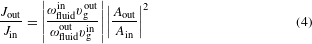To estimate this factor, we assume that the flow varies slowly in the observation window, such that ωfluid obeys the usual dispersion relation of water waves, ωfluid2 = gktanh(h0k). (This amounts to a Wentzel–Kramers–Brillouin (WKB) approximation, and capillarity is neglected.) Under this assumption, the group velocity is the sum of the group velocity in the fluid frame, given by the dispersion relation,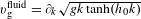, and the radial velocity of the flow vr. Hence the group velocity needed for the energy ratio equation (4) splits into two: vg = vgfluid + vr. The first term is obtained only with the values of kin and kout, extracted from the radial Fourier profiles. The second term requires the value of vr, which we do not have to a sufficient accuracy. However, using the PIV data, we see that the contribution of this last term amounts to less than 1% in all experiments (this uncertainty is added to the error bars on Figs 2 and 3).

### Data analysis.

We record the free surface of the water in a region of 1.33 m × 0.98 m over the vortex during 13.2 s. From the sensor we obtain 248 reconstructions of the free surface. These reconstructions are triplets Xij, Yij and Zij giving the coordinates of 640 × 480 points on the free surface. Because of the shape of the vortex, and noise, parts of the free surface cannot be seen by our sensor, resulting in black spots on the image. Isolated black spots are corrected by interpolating the value of the height using their neighbours. This procedure is not possible in the core of the vortex, and we set these values to zero.

To filter the signal in frequency, we first crop the signal in time so as to keep an integer number of cycles to reduce spectral leakage. We then select a single frequency corresponding to the excitation frequency f0. After this filter, we are left with a two-dimensional array of complex values, encoding the fluctuations of the water height ξ(Xij, Yij) at the frequency f0. ξ(Xij, Yij) is defined on the grids Xij and Yij, whose points are not perfectly equidistant (this is due to the fact that the discretization is done by the sensor software in a coordinate system that is not perfectly parallel to the free surface).

To select specific azimuthal numbers, we convert the signal from Cartesian to polar coordinates. For this we need to find the centre of symmetry of the background flow. We define our centre to be the centre of the shadow of the vortex, averaged over time (the fluctuations in time are smaller than a pixel). To verify that this choice does not affect the end result, we performed a statistical analysis on different centre choices around this value, and added the standard deviation to the error bars. Once the centre is chosen, we perform a discrete Fourier transform on the irregular grid (Xij, Yij). We create an irregular polar grid (rij, θij) and we compute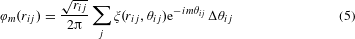where Δθij = (ΔXijΔYij)/(rijΔrij) is the line element along a circle of radius rij.

To extract the inward and outward amplitudes Ain and Aout, we compute the radial Fourier transform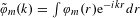over the window [rmin, rmax]. Due to the size of the window compared to the wavelength of the waves, we can capture only a few oscillations in the radial direction, typically between 1 and 3. This results in broad peaks around the values kin and kout of the inward and outward components. We assume that these peaks contain only one wavelength (no superposition of nearby wavelengths), which is corroborated by the fact that we have filtered in time, and the dispersion relation imposes a single wavelength at a given frequency. To reduce spectral leakage, we use a Hamming window function on [rmin, rmax], defined as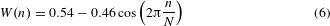where n is the pixel index running from 1 to N. This window is optimized to reduce the secondary lobe, and allows us to better distinguish peaks with different amplitudes30. In Supplementary Fig. 1, we show the radial Fourier profiles for various m for a typical experiment (left column), and the raw radial profiles and how they are approximated by equation (2) (right column).

We also extracted the signal-to-noise ratio by comparing the standard deviation of the noise to the value of our signal. It is sufficiently high to exclude the possibility that the amplification we observed is due to a noise fluctuation, and its contribution is negligible compared to other sources of error.

### PIV measurements.

Close to the vortex core, the draining bathtub vortex is cylindrically symmetric to a good approximation. An appropriate choice of coordinates is, therefore, cylindrical coordinates (r, θ, z). The velocity field will be independent of the angle θ and can be expressed as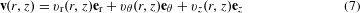We are specifically interested in the velocity field at the free surface z = h(r). When the free surface is flat, h is constant and the vertical velocity vz vanishes. When the surface is not flat, the vz component can be deduced from vr using the free surface profile h(r) and the equation vz(r, h(r)) = (rh)vr |z=h. To obtain an estimate of vz, we use a simple model for the free surface shape31,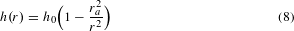where h0 is the water height far from the vortex and ra is the radial position at which the free surface passes through the sink hole. This approximation captures the essential features of our experimental data. The components vr and vθ are determined through the technique of particle imaging velocimetry (PIV), implemented through the Matlab extension PIVlab32,33. The technique can be summarized as follows.

The flow is seeded with flat paper particles of mean diameter d = 2 mm. The particles are buoyant, which allows us to evaluate the velocity field exclusively at the free surface. The amount by which a particle deviates from the streamlines of the flow is given by the velocity lag Us = d2(ρρ0)a/18μ (ref. 32), where ρ is the density of a particle, ρ0 is the density of water, μ is the dynamic viscosity of water and a is the acceleration of a particle. For fluid accelerations in our system this is at most of the order 10−4 m s−1, an order of magnitude below the smallest velocity in the flow. Thus we can safely neglect the effects of the velocity lag when considering the motions of the particles in the flow.

The surface is illuminated using two light panels positioned at opposite sides of the tank. The flow is imaged from above using a Phantom Miro Lab 340 high-speed camera at a frame rate of 800 fps for an exposure time of 1,200 μs. The raw images are analysed using PIVlab by taking a small window in one image and looking for a window within the next image which maximizes the correlation between the two. By knowing the distance between these two windows and the time step between two images, it is possible to give each point on the image a velocity vector. This process is repeated for all subsequent images, and the results are then averaged in time to give a mean velocity field.

The resulting velocity field is decomposed onto an (r, θ)-basis centred about the vortex origin to give the components vr and vθ. The centre is chosen so as to maximize the symmetry. In Fig. 4b we show the norm of the velocity field on the free surface. We see that our vortex flow is symmetric to a good approximation. To quantify the asymmetry of the flow, we estimate the coupling of waves with mm′ through asymmetry. The change of the reflection coefficient due to this coupling is of the order of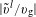, where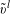is the angular Fourier component of azimuthal number l = mm′. This ratio is smaller than 3% in all experiments. To obtain the radial profiles of vr and vθ, we integrate them over the angle θ. In Figs 4c, d we show vθ and the inward velocity tangent to the free surface,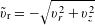, as functions of r.

We compare the data for vθ with the Lamb vortex31,where Ω0 is the maximum angular velocity in the rotational core of characteristic radius r0. (For vθ we have Ω0 = 69.4 rad s−1 and r0 = 1.34 cm, and for vr we have Ω0 = −4.52 rad s−1 and r0 = 1.39 cm.) Outside the vortex core, this model reduces to the characteristic 1/r dependence of an incompressible, irrotational flow depending only on r. By observing that vθ and vr exhibit similar qualitative behaviour, vr is also fitted with a model of the form of equation (9). Figure 4c, d show that equation (9) captures the essential features of the measured velocity profiles. The angular velocity of the flow is given by Ω(r) = vθ/r which is shown in Fig. 4a. From this plot it is clear that Ω reaches large enough values to be consistent with the detection of superradiance.

### Data availability.

The data that support the plots within this paper and other findings of this study are available from the corresponding author upon reasonable request.